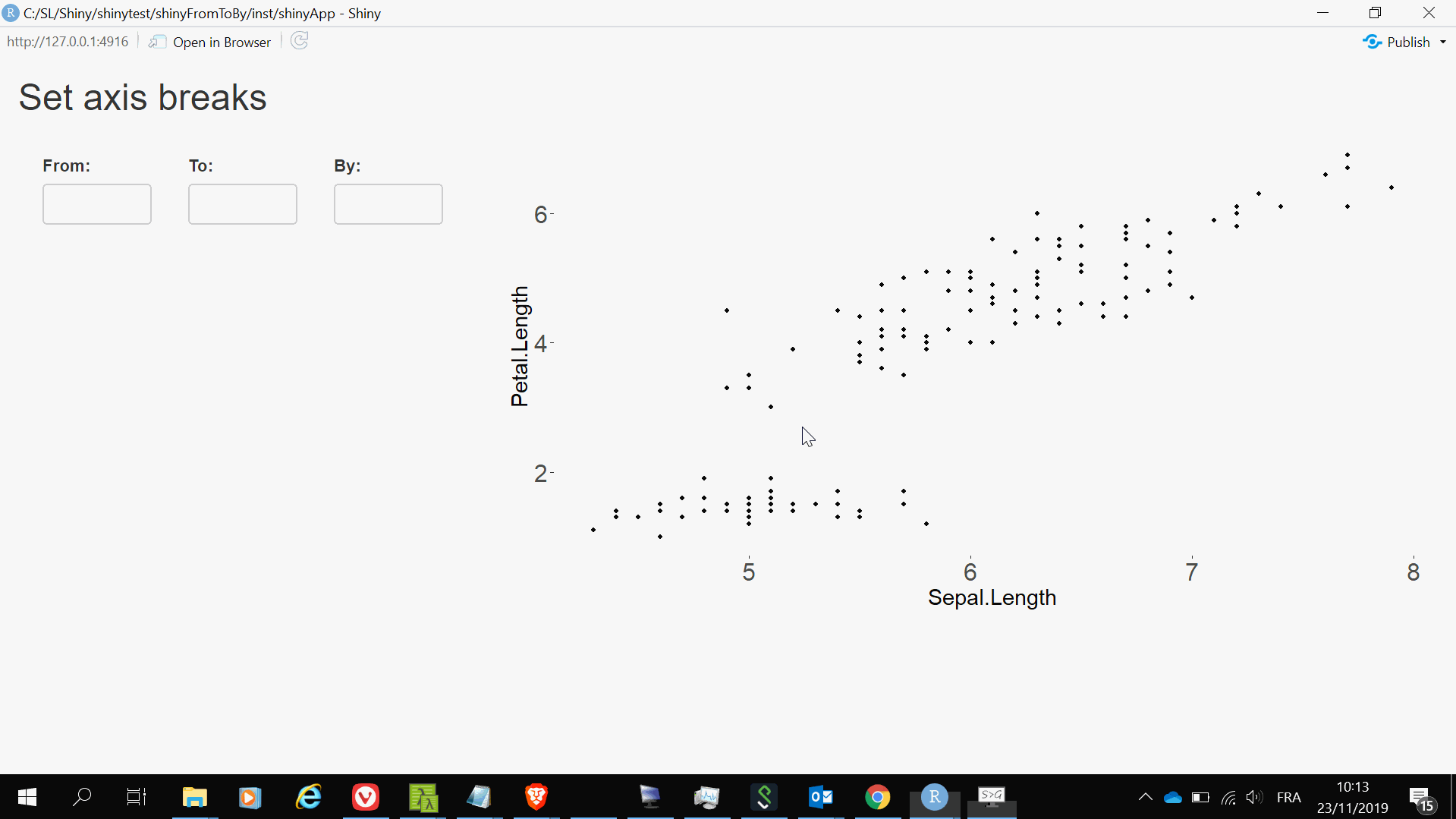Want to share your content on R-bloggers? click here if you have a blog, or here if you don't.

## The shiny app

Here is a simple shiny app:

```library(shiny)
library(ggplot2)

# UI ####
ui <- fluidPage(

titlePanel("Set axis breaks"),

sidebarLayout(
# sidebar ####
sidebarPanel(
fluidRow(
column(
width = 4,
numericInput("from", "From:", value = NULL)
),
column(
width = 4,
numericInput("to", "To:", value = NULL)
),
column(
width = 4,
numericInput("by", "By:", value = NULL)
)
)
),

# main panel ####
mainPanel(
plotOutput("ggplot")
)
)
)

# SERVER ####
server <- function(input, output) {

FromToBy <- reactiveVal()

observe({
breaks <- tryCatch({
seq(input[["from"]], input[["to"]], input[["by"]])
}, error = function(e) NULL)
FromToBy(breaks)
})

scatterplot <- reactive({
gg <- ggplot(iris, aes(Sepal.Length, Petal.Length)) + geom_point()
if(!is.null(FromToBy())){
gg <- gg + scale_y_continuous(breaks = FromToBy())
}
gg + theme(
axis.text = element_text(size = 20),
axis.title = element_text(size = 18)
)
})

output[["ggplot"]] <- renderPlot({
scatterplot()
})

observe({ # this is for shinytest - see later ####
req(scatterplot())
ggg <- ggplot_build(scatterplot())
exportTestValues(panelParams = ggg\$layout\$panel_params[[1L]])
})

}

# Run the application ####
shinyApp(ui = ui, server = server)```

It allows to set the breaks of the y-axis of the graph:## Unit test

Say we want to test whether the app correctly sets the axis breaks.

When you have a `ggplot`, you can get the y-axis breaks as follows:

```library(ggplot2)
gg <- ggplot(iris, aes(Sepal.Length, Petal.Length)) + geom_point()
ggg <- ggplot_build(gg)
ggg\$layout\$panel_params[[1L]][["y.major_source"]]
##  2 4 6```

With this piece of code of the app:

```observe({ # this is for shinytest - see later ####
req(scatterplot())
ggg <- ggplot_build(scatterplot())
exportTestValues(panelParams = ggg\$layout\$panel_params[[1L]])
})```

we export `ggg\$layout\$panel_params[[1L]]`.

Assume our package is named `shinyFromToBy` and the shiny app is saved in file `app.R` in the `inst/shinyApp` folder. Then, here is our unit test (to put in the `tests/testthat` folder):

```context("y-axis breaks")

test_that("y-breaks are correct", {

skip_if_not_installed("shinytest")
library(shinytest)

app <- ShinyDriver\$new(
system.file("shinyApp", package = "shinyFromToBy"),
shinyOptions = list(test.mode = TRUE)
)
app\$setInputs(from = 1)
app\$setInputs(to = 7)
app\$setInputs(by = 0.5)
panelParams <- app\$getAllValues()\$export[["panelParams"]]

expect_identical(
panelParams[["y.major_source"]],
seq(1, 7, by = 0.5)
)

app\$stop()

})```